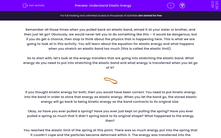# Understand Elastic Energy

In this worksheet, students will look at how energy can be stored elastically and how to calculate such energy. They will not cover Hookes law or springs in this activity, for this please refer to our worksheet titled 'Understand Forces and Elasticity'.Key stage:  KS 4

Year:  GCSE

GCSE Boards:   AQA, AQA Trilogy, AQA Synergy, OCR 21st Century, OCR Gateway, Eduqas,

Popular topics:   Physics worksheets

Difficulty level:#### Worksheet Overview

Remember all those times when you pulled back an elastic band, aimed it at your sister or brother, and then just let go? Obviously, we would never tell you to do something like this – it would be dangerous, but if you do get a chance, then stop to think about the physics that is happening here. This is what we are going to look at in this activity. You will learn about the equation for elastic energy and what happens when you stretch an elastic band too much (this is called the elastic limit).

So to start with, let’s look at the energy transfers that are going into stretching the elastic band. What energy do you need to put into stretching the elastic band and what energy is transferred when you let go of it?If you thought kinetic energy for both, then you would have been correct. You need to put kinetic energy into the band in order to store that energy as elastic energy. When you let the band go, the stored elastic energy will go back to being kinetic energy as the band contracts to its original size.

Okay, so have you ever pulled a spring? Have you ever just kept on pulling the spring? Have you ever pulled a spring so much that it didn’t spring back to its original shape? What happened to the energy then?

You reached the elastic limit of the spring at this point. There was so much energy put into the spring that it couldn’t cope and the particles became deformed within it. The energy was transferred into the particles in order to rearrange them – it’s not gone, but it is really difficult to get back - the spring is essentially broken.Now it’s time for the part that you have all been waiting for – the maths!

Here is the equation for elastic energy:

E = ½kx²

E =  elastic energy in joules (J)
k = the spring constant in newtons per metre (N/m)  Each spring will have its own spring constant which tells us how much energy a spring can store. A higher spring constant means a spring can store more energy.
x = extension in metres (m). How far the spring has moved from its original size.

Let’s have a go at a question now:

Jean is doing an experiment with springs. He starts with a spring of length 0.010 m and places masses on it until it reaches a length of 0.015 m. The box of springs says that the spring constant is 200 N/m. Calculate the energy put into the spring from this extension.

Step 1   Find all the numbers and highlight them:

Jean is doing an experiment with springs. He starts with a spring of length 0.010 m and places masses on it until it reaches a length of 0.015 m. The box of springs says that the spring constant is 200 N/m. Calculate the energy put into the spring from this extension.

Step 2   Write out the numbers next to their symbols:

E = ?
k = 200 N/m
x = 0.015 – 0.010 = 0.005 m
We needed to work out the change in x because x is the change in length (extension) of the spring. So, all you do is take the smallest value from the largest value.

Step 3   Put these numbers into the equation:

E = (0.5 x 200) x (0.0052)

Step 4   Do the parts in the brackets first:

E = 100 x 0.000025

Step 5   Do the rest of the equation!

E = 0.0025 J

DO NOT forget the units!

Are you ready for some questions now?

### What is EdPlace?

We're your National Curriculum aligned online education content provider helping each child succeed in English, maths and science from year 1 to GCSE. With an EdPlace account you’ll be able to track and measure progress, helping each child achieve their best. We build confidence and attainment by personalising each child’s learning at a level that suits them.

Get started